# Verify that the given function is a solution to the given differential equation (c1 and c2...

Verify that the given function is a solution to the given differential equation (c1 and c2 are arbitrary constants), and state the maximum interval over which the solution is valid.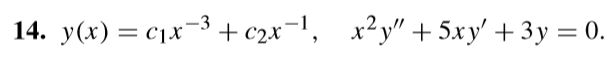14. y(x) = cix-3 + c2x-1, x2y" + 5xy' + 3y = 0.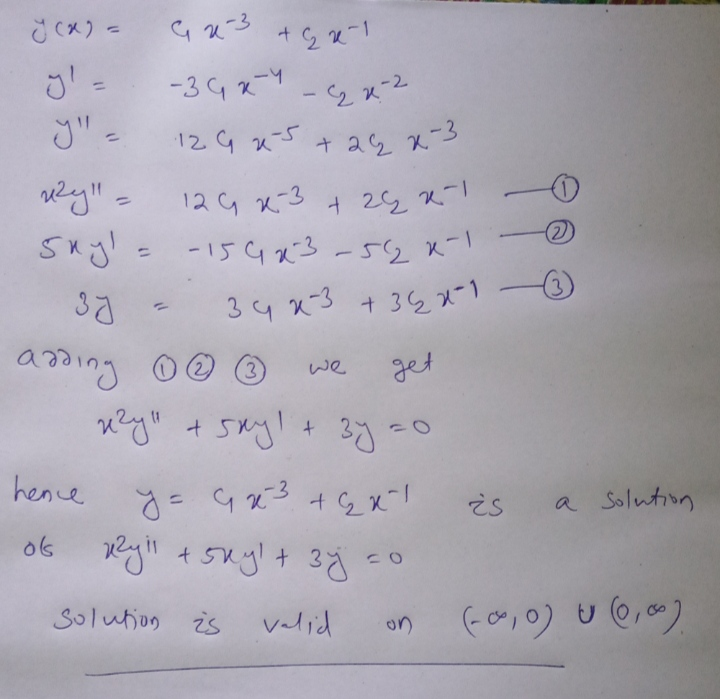I use differenriation to solve this problem

##### Add Answer of: Verify that the given function is a solution to the given differential equation (c1 and c2...
Similar Homework Help Questions
• ### Verify that the given function is a solution to the given differential equation (c1 and c2...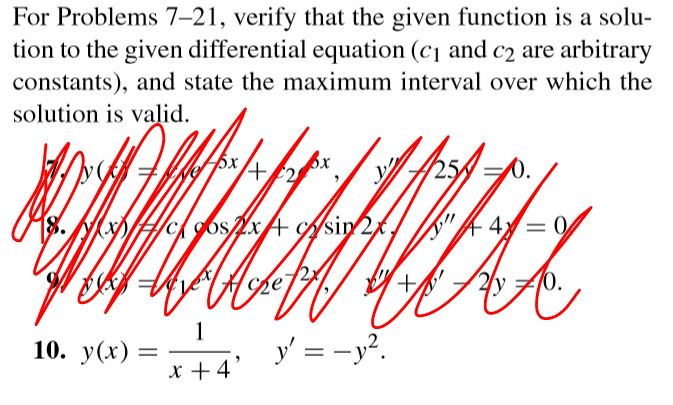Verify that the given function is a solution to the given differential equation (c1 and c2 are arbitrary constants) and state the maximum interval over which the solution is valid. For Problems 7-21, verify that the given function is a solu- tion to the given differential equation (cy and c2 are arbitrary constants), and state the maximum interval over which the solution is valid. ya Sx +42 25 WID#cigos x A Asin 2%, = 0 BAWK vel Hope 2y +10....

• ### verify that the given function is a solution to the given differential equation (c1 andc2 arbitrary...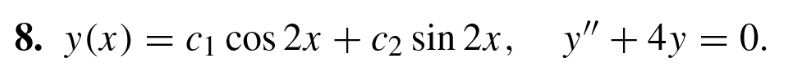verify that the given function is a solution to the given differential equation (c1 andc2 arbitrary constants), and state the maximum interval over which the solution is valid. 8. y(x) = cj cos 2x + c2 sin 2x, y + 4y = 0.

• ### Question 2: Differential Equations a) (3 points) Find the general solution to the equation. Use C,C1,C2...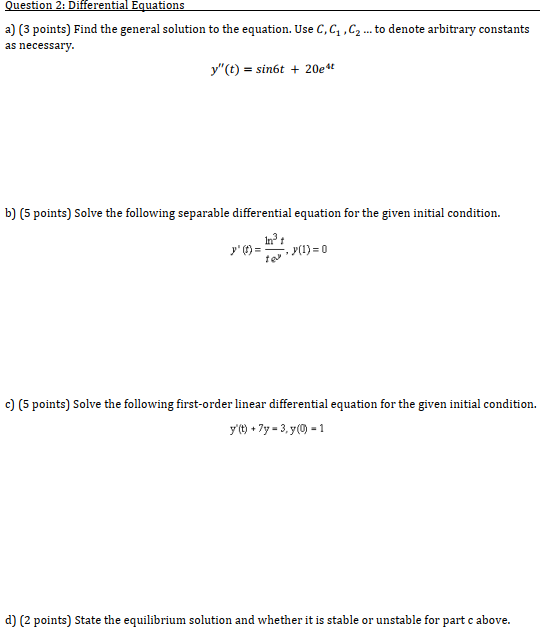Question 2: Differential Equations a) (3 points) Find the general solution to the equation. Use C,C1,C2 ... to denote arbitrary constants as necessary. y"(t) = sin6t + 20e b) (5 points) Solve the following separable differential equation for the given initial condition. y')= (1) = 0 c) (5 points) Solve the following first-order linear differential equation for the given initial condition. y't) + 7y - 3,y(0) - 1 d) (2 points) State the equilibrium solution and whether it is stable...

• ### Differential Equation

a) Compute y’ and y’ and then combine these derivatives with y as a linear second-order differential equation that is free of the symbols c1 and c2 and has theform F(y, y’, y’’) = 0. The symbols c1 and c2 represent constants. y = c1ex + c2xexb) Verify that the indicated function (below) is a particular solution of the given differential equation. Give an interval of definition I for eachsolution.y’’ + y = sec x; y = x sin x...

• ### Find the general solution to the given differential equation and the maximum interval on which the...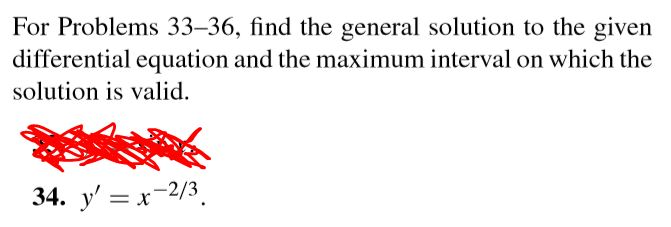Find the general solution to the given differential equation and the maximum interval on which the solution is valid. y' = x^(-2/3) For Problems 33–36, find the general solution to the given differential equation and the maximum interval on which the solution is valid. 34. y' = x-2/3

• ### Answer all Please! Thanks 1. Confirming Solutions to Differential Equations: Verify that each function does in fact solve the given differential equation. If there are parameters in the function (A....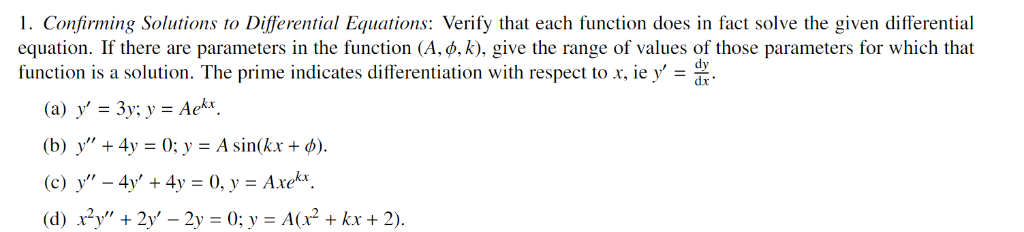Answer all Please! Thanks 1. Confirming Solutions to Differential Equations: Verify that each function does in fact solve the given differential equation. If there are parameters in the function (A. b. k), give the range of values of those parameters for which that function is a solution. The prime indicates differentiation with respect od dr' (b) y" + 4y = 0; y = A sin(kx + φ). (c) y"-4s, + 4y = 0, y = Axe . (d) x2y', +...

• ### (8 pts) In this problem you will solve the non-homogeneous differential equation y" + 9y =...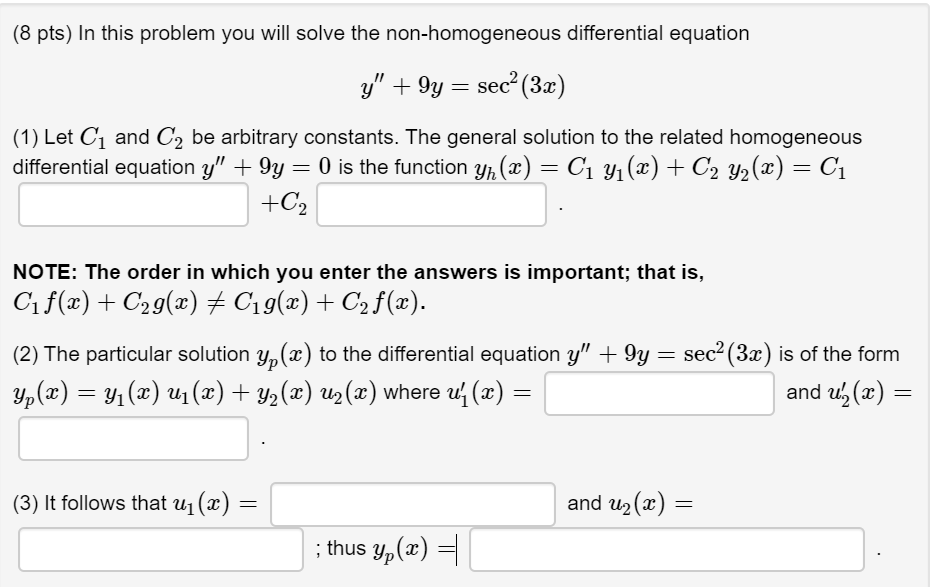(8 pts) In this problem you will solve the non-homogeneous differential equation y" + 9y = sec (3x) (1) Let C and C2 be arbitrary constants. The general solution to the related homogeneous differential equation y" + 9y = 0 is the function yn (x) = C1 yı(2) + C2 y2(x) = C1 +C2 NOTE: The order in which you enter the answers is important; that is, Cif(x) + C2g(x) + C19(x) + C2 f(x). (2) The particular solution yp(x)...

• ### II. Determine the general solution of the given 2nd order linear homogeneous equation. 1. y" -...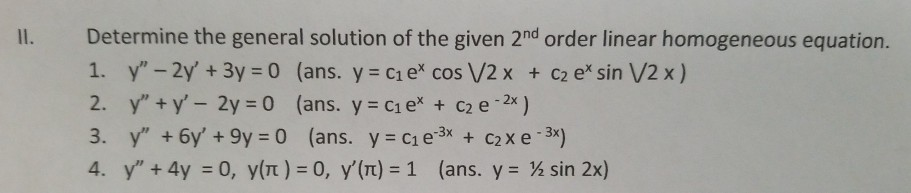II. Determine the general solution of the given 2nd order linear homogeneous equation. 1. y" - 2y' + 3y = 0 (ans. y = ci e' cos V2 x + C2 e* sin V2 x) 2. y" + y' - 2y = 0 (ans. y = C1 ex + C2 e -2x) 3. y" + 6y' + 9y = 0 (ans. y = C1 e 3x + c2x e-3x) 4. Y" + 4y = 0, y(t) = 0, y'(T) =...

• ### Problem 5: Find the general solution to the following differential equation using the method of variation...

Problem 5: Find the general solution to the following differential equation using the method of variation of parameters: x2y"+ xy' + (x2− 1/4 )y = x 3/2 given that the complementary solution on (0,∞) is given by yc = c1x-1/2cos(x) + c2x -1/2sin(x).

• ### Differential equation 1. Chapter 4 covers differential equations of the form an(x)y("4a-,(x)ye-i) + +4(x)y'+...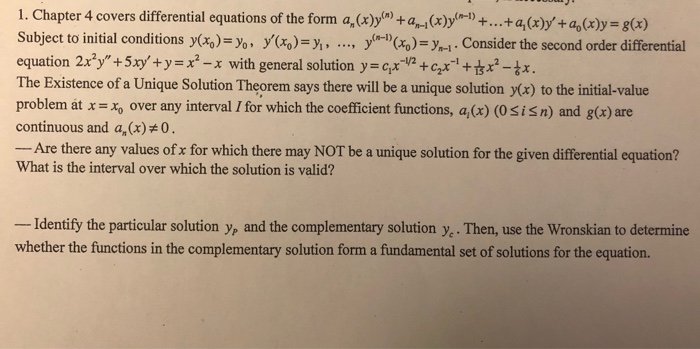Differential equation 1. Chapter 4 covers differential equations of the form an(x)y("4a-,(x)ye-i) + +4(x)y'+4(x)-g(x) Subject to initial conditions y)oyy-Co) Consider the second order differential equation 2x2y" + 5xy, + y-r-x 2- The Existence of a Unique Solution Theorem says there will be a unique solution y(x) to the initial-value problem at x=而over any interval 1 for which the coefficient functions, ai (x) (0 S is n) and g(x) are continuous and a, (x)0. Are there any values of x for...

Free Homework App